Next: Extended-system Adaptive Biasing Force Up: Biasing and analysis methods Previous: Biasing and analysis methods   Contents   Index

Subsections

For a full description of the Adaptive Biasing Force method, see reference . For details about this implementation, see references  and . When publishing research that makes use of this functionality, please cite references  and .

An alternate usage of this feature is the application of custom tabulated biasing potentials to one or more colvars. See inputPrefix and updateBias below.

Combining ABF with the extended Lagrangian feature (13.2.4) of the variables produces the extended-system ABF variant of the method (13.5.2).

ABF is based on the thermodynamic integration (TI) scheme for computing free energy profiles. The free energy as a function of a set of collective variables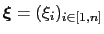is defined from the canonical distribution of,: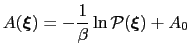(13.14)

In the TI formalism, the free energy is obtained from its gradient, which is generally calculated in the form of the average of a force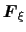exerted on, taken over an iso-surface:(13.15)

Several formulae that take the form of (13.16) have been proposed. This implementation relies partly on the classic formulation , and partly on a more versatile scheme originating in a work by Ruiz-Montero et al. , generalized by den Otter  and extended to multiple variables by Ciccotti et al. . Consider a system subject to constraints of the form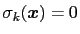. Let (be arbitrarily chosen vector fields () verifying, for all,, and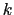: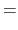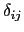(13.16)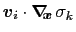0 (13.17)

then the following holds :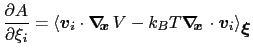(13.18)

where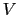is the potential energy function.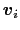can be interpreted as the direction along which the force acting on variableis measured, whereas the second term in the average corresponds to the geometric entropy contribution that appears as a Jacobian correction in the classic formalism . Condition (13.17) states that the direction along which the total force onis measured is orthogonal to the gradient of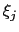, which means that the force measured ondoes not act on.

Equation (13.18) implies that constraint forces are orthogonal to the directions along which the free energy gradient is measured, so that the measurement is effectively performed on unconstrained degrees of freedom.

In the framework of ABF,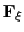is accumulated in bins of finite size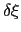, thereby providing an estimate of the free energy gradient according to equation (13.16). The biasing force applied along the collective variables to overcome free energy barriers is calculated as: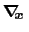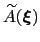(13.19)

wheredenotes the current estimate of the free energy gradient at the current pointin the collective variable subspace, andis a scaling factor that is ramped from 0 to 1 as the local number of samplesincreases to prevent nonequilibrium effects in the early phase of the simulation, when the gradient estimate has a large variance. See the fullSamples parameter below for details.

As sampling of the phase space proceeds, the estimateis progressively refined. The biasing force introduced in the equations of motion guarantees that in the bin centered around, the forces acting along the selected collective variables average to zero over time. Eventually, as the undelying free energy surface is canceled by the adaptive bias, evolution of the system alongis governed mainly by diffusion. Although this implementation of ABF can in principle be used in arbitrary dimension, a higher-dimension collective variable space is likely to result in sampling difficulties. Most commonly, the number of variables is one or two.

### ABF requirements on collective variables

The following conditions must be met for an ABF simulation to be possible and to produce an accurate estimate of the free energy profile. Note that these requirements do not apply when using the extended-system ABF method (13.5.2).

1. Only linear combinations of colvar components can be used in ABF calculations.
2. Availability of total forces is necessary. The following colvar components can be used in ABF calculations: distance, distance_xy, distance_z, angle, dihedral, gyration, rmsd and eigenvector. Atom groups may not be replaced by dummy atoms, unless they are excluded from the force measurement by specifying oneSiteTotalForce, if available.
3. Mutual orthogonality of colvars. In a multidimensional ABF calculation, equation (13.17) must be satisfied for any two colvarsand. Various cases fulfill this orthogonality condition:
•andare based on non-overlapping sets of atoms.
• atoms involved in the force measurement ondo not participate in the definition of. This can be obtained using the option oneSiteTotalForce of the distance, angle, and dihedral components (example: Ramachandran angles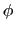,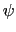).
•andare orthogonal by construction. Useful cases are the sum and difference of two components, or distance_z and distance_xy using the same axis.
4. Mutual orthogonality of components: when several components are combined into a colvar, it is assumed that their vectors(equation (13.19)) are mutually orthogonal. The cases described for colvars in the previous paragraph apply.
5. Orthogonality of colvars and constraints: equation 13.18 can be satisfied in two simple ways, if either no constrained atoms are involved in the force measurement (see point 3 above) or pairs of atoms joined by a constrained bond are part of an atom group which only intervenes through its center (center of mass or geometric center) in the force measurement. In the latter case, the contributions of the two atoms to the left-hand side of equation 13.18 cancel out. For example, all atoms of a rigid TIP3P water molecule can safely be included in an atom group used in a distance component.

### Parameters for ABF

ABF depends on parameters from collective variables to define the grid on which free energy gradients are computed. In the direction of each colvar, the grid ranges from lowerBoundary to upperBoundary, and the bin width (grid spacing) is set by the width parameter (see 13.2.1). The following specific parameters can be set in the ABF configuration block:

• name: see definition of name (biasing and analysis methods)
• colvars: see definition of colvars (biasing and analysis methods)

• fullSamplesNumber of samples in a bin prior to application of the ABF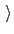Context: abf
Acceptable values: positive integer
Default value: 200
Description: To avoid nonequilibrium effects due to large fluctuations of the force exerted along the colvars, it is recommended to apply a biasing force only after a the estimate has started converging. If fullSamples is non-zero, the applied biasing force is scaled by a factorbetween 0 and 1. If the number of samplesin the current bin is higher than fullSamples, the factor is one. If it is less than half of fullSamples, the factor is zero and no bias is applied. Between those two thresholds, the factor follows a linear ramp from 0 to 1: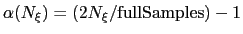.

• maxForceMaximum magnitude of the ABF forceContext: abf
Acceptable values: positive decimals (one per colvar)
Default value: disabled
Description: This option enforces a cap on the magnitude of the biasing force effectively applied by this ABF bias on each colvar. This can be useful in the presence of singularities in the PMF such as hard walls, where the discretization of the average force becomes very inaccurate, causing the colvar's diffusion to get stuck'' at the singularity. To enable this cap, provide one non-negative value for each colvar. The unit of force is kcal/mol divided by the colvar unit.

• hideJacobianRemove geometric entropy term from calculated free energy gradient?Context: abf
Acceptable values: boolean
Default value: no
Description: In a few special cases, most notably distance-based variables, an alternate definition of the potential of mean force is traditionally used, which excludes the Jacobian term describing the effect of geometric entropy on the distribution of the variable. This results, for example, in particle-particle potentials of mean force being flat at large separations. Setting this parameter to yes causes the output data to follow that convention, by removing this contribution from the output gradients while applying internally the corresponding correction to ensure uniform sampling. It is not allowed for colvars with multiple components.

• outputFreqFrequency (in timesteps) at which ABF data files are refreshedContext: abf
Acceptable values: positive integer
Default value: Colvars module restart frequency
Description: The files containing the free energy gradient estimate and sampling histogram (and the PMF in one-dimensional calculations) are written on disk at the given time interval.

• historyFreqFrequency (in timesteps) at which ABF history files are accumulatedContext: abf
Acceptable values: positive integer
Default value: 0
Description: If this number is non-zero, the free energy gradient estimate and sampling histogram (and the PMF in one-dimensional calculations) are appended to files on disk at the given time interval. History file names use the same prefix as output files, with .hist'' appended.

• inputPrefixFilename prefix for reading ABF dataContext: abf
Acceptable values: list of strings
Description: If this parameter is set, for each item in the list, ABF tries to read a gradient and a sampling files named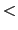inputPrefix.grad andinputPrefix.count. This is done at startup and sets the initial state of the ABF algorithm. The data from all provided files is combined appropriately. Also, the grid definition (min and max values, width) need not be the same that for the current run. This command is useful to piece together data from simulations in different regions of collective variable space, or change the colvar boundary values and widths. Note that it is not recommended to use it to switch to a smaller width, as that will leave some bins empty in the finer data grid. This option is NOT compatible with reading the data from a restart file (cv load command).

• applyBiasApply the ABF bias?Context: abf
Acceptable values: boolean
Default value: yes
Description: If this is set to no, the calculation proceeds normally but the adaptive biasing force is not applied. Data is still collected to compute the free energy gradient. This is mostly intended for testing purposes, and should not be used in routine simulations.

• updateBiasUpdate the ABF bias?Context: abf
Acceptable values: boolean
Default value: yes
Description: If this is set to no, the initial biasing force (e.g. read from a restart file or through inputPrefix) is not updated during the simulation. As a result, a constant bias is applied. This can be used to apply a custom, tabulated biasing potential to any combination of colvars. To that effect, one should prepare a gradient file containing the gradient of the potential to be applied (negative of the bias force), and a count file containing only values greater than fullSamples. These files must match the grid parameters of the colvars.

### Output files

The ABF bias produces the following files, all in multicolumn text format:

• outputName.grad: current estimate of the free energy gradient (grid), in multicolumn;
• outputName.count: histogram of samples collected, on the same grid;
• outputName.pmf: only for one-dimensional calculations, integrated free energy profile or PMF.

If several ABF biases are defined concurrently, their name is inserted to produce unique filenames for output, as in outputName.abf1.grad. This should not be done routinely and could lead to meaningless results: only do it if you know what you are doing!

If the colvar space has been partitioned into sections (windows) in which independent ABF simulations have been run, the resulting data can be merged using the inputPrefix option described above (a run of 0 steps is enough).

### Post-processing: reconstructing a multidimensional free energy surface

If a one-dimensional calculation is performed, the estimated free energy gradient is automatically integrated and a potential of mean force is written under the file name <outputName>.pmf, in a plain text format that can be read by most data plotting and analysis programs (e.g. gnuplot).

In dimension 2 or greater, integrating the discretized gradient becomes non-trivial. The standalone utility abf_integrate is provided to perform that task. abf_integrate reads the gradient data and uses it to perform a Monte-Carlo (M-C) simulation in discretized collective variable space (specifically, on the same grid used by ABF to discretize the free energy gradient). By default, a history-dependent bias (similar in spirit to metadynamics) is used: at each M-C step, the bias at the current position is incremented by a preset amount (the hill height). Upon convergence, this bias counteracts optimally the underlying gradient; it is negated to obtain the estimate of the free energy surface.

abf_integrate is invoked using the command-line:
abf_integrate <gradient_file> [-n <nsteps>] [-t <temp>] [-m (0|1)] [-h <hill_height>] [-f <factor>]

The gradient file name is provided first, followed by other parameters in any order. They are described below, with their default value in square brackets:

• -n: number of M-C steps to be performed; by default, a minimal number of steps is chosen based on the size of the grid, and the integration runs until a convergence criterion is satisfied (based on the RMSD between the target gradient and the real PMF gradient)
• -t: temperature for M-C sampling (unrelated to the simulation temperature) [500 K]
• -m: use metadynamics-like biased sampling? (0 = false) 
• -h: increment for the history-dependent bias (hill height'') [0.01 kcal/mol]
• -f: if non-zero, this factor is used to scale the increment stepwise in the second half of the M-C sampling to refine the free energy estimate [0.5]

Using the default values of all parameters should give reasonable results in most cases.

abf_integrate produces the following output files:

• <gradient_file>.pmf: computed free energy surface
• <gradient_file>.histo: histogram of M-C sampling (not usable in a straightforward way if the history-dependent bias has been applied)
• <gradient_file>.est: estimated gradient of the calculated free energy surface (from finite differences)
• <gradient_file>.dev: deviation between the user-provided numerical gradient and the actual gradient of the calculated free energy surface. The RMS norm of this vector field is used as a convergence criteria and displayed periodically during the integration.

Note: Typically, the deviation'' vector field does not vanish as the integration converges. This happens because the numerical estimate of the gradient does not exactly derive from a potential, due to numerical approximations used to obtain it (finite sampling and discretization on a grid).Next: Extended-system Adaptive Biasing Force Up: Biasing and analysis methods Previous: Biasing and analysis methods   Contents   Index
vmd@ks.uiuc.edu